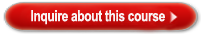### Oracle - Course Details

OraS:E Oracle Spatial: EssentialsOracle Course Description:
The course extensively covers the concepts and usage of the native data types, functions and operators available in Oracle Spatial for implementing geospatial applications and location-based services.
Oracle Course Duration:
5 DAYS
Oracle Course Target Audience:
Application Developers,PL/SQL Developer,Technical Administrator,Technical Consultant
Oracle Course Prerequisite:
Familiarity with SQL (recommended OU course: Introduction to SQL),Familiarity with Object relational data model,Familiarity with PL/SQL (recommended OU course: Program with PL/SQL),Familiarity with mathematical geometry concepts
Course Content:
• Introduction

• Oracle Database: Location Features
• Oracle Spatial: Spatial Data Management for Enterprise Applications
• Oracle Spatial Development History
• Oracle Spatial and Locator: art of the Oracle DBMS Kernel
• Oracle Spatial Object-Relational Model
• Review: Oracle’s Object-Relational Model
• Common Geographical Terms Used in the Course
• Oracle Spatial Documentation and Resources

• Overview of Oracle Spatial Concepts

• Define Oracle Spatial
• Describe Geometric Primitive Types
• Describe the Spatial Data Model
• Coordinate Systems: Concepts
• Explain Spatial Indexing
• Describe the Optimized Query Model
• Define Linear Referencing System
• Define Geocoding and Routing

• Creating Spatial Layers

• Describe the MDSYS Schema
• Spatial Native Data Type: SDO_GEOMETRY
• Define different types of geometry elements
• Construction of geometries by using the INSERT statement
• Manage Spatial metadata

• Defining Collection Geometries

• Define Collection geometries: Multipoint, Multiline string, and Multipolygon
• Describe Oriented point
• SDO_GEOMETRY constructors and member methods

• Associating Spatial Layers with Coordinate Systems

• Define Coordinate systems and their different types
• Geodetic coordinate system concepts
• Whole earth geometry model and tolerance
• Coordinate system transformations
• Units supported by Oracle Spatial

• Different ways of loading spatial data
• Export and import utilities of the Data Pump technology
• Load data using transportable tablespace
• Load data using transactional insert
• Use the Java shapefile converter

• Validating and Debugging Geometries

• Validation functions: SDO_GEOM.VALIDATE_GEOMETRY_WITH_CONTEXT and SDO_GEOM.VALIDATE_LAYER_WITH_CONTEXT
• Geometry debugging functions: SDO_UTIL.GETVERTICES, SDO_UTIL.RECTIFY_GEOMETRY, and SDO_UTIL.EXTRACT
• Strategy for Geometry Validation

• Using the Oracle Application Server MapViewer

• Introduction to MapViewer
• Architecture of Oracle Application Server MapViewer
• Installation of Oracle Application Server MapViewer
• Use MapViewer demos
• Edit MapViewer Configuration File

• Indexing Spatial Data

• Concepts of R-tree indexing
• CREATE INDEX and the R-tree parameters
• Analyze, drop, and alter operations on the spatial index
• Use the Spatial index dictionary views
• Estimate R-tree index size and the resources required

• Querying Spatial Data

• Overview of the Spatial query model
• Overview of spatial operators, procedures, and functions
• Use the SDO_FILTER operator
• Define Spatial topological relationships
• Use the SDO_RELATE operator
• Use the SDO_GEOM.RELATE function

• Using SDO_WITHIN_DISTANCE, SDO_NN, and SDO_JOIN Operators

• Spatial queries and operators
• Describe the SDO_WITHIN_DISTANCE operator
• Describe the SDO_NN operator
• Spatial join by using the SDO_JOIN operator

• Analyzing Geometries by Using Spatial Operators and Functions

• Calculation of the area, length, and distance between geometries
• Describe Arc densification and buffering
• Use the Spatial Boolean functions
• Explicit transformations with spatial functions

• Using Spatial Analysis, MBR, Utility, and Aggregate Functions

• Describe some of the Spatial analysis functions
• Describe some of the Spatial MBR functions
• Describe some of the Spatial utility functions
• Describe some of the Spatial aggregate functions
• Conversion between SDO_GEOMETRY and Geography Markup Language (GML)

• Defining Maps by Using the Map Builder Tool

• Introduction to Map Builder
• Export and import styles
• Use of Map Builder to administer style, theme, and map definitions
• Use the Sample mapclient.jsp
• Define a Sample XML request with elements
• Open Geospatial Consortium (OGC) Web Map Service (WMS) and Oracle Workspace Manager support

• Leveraging Oracle Maps: The Map Cache and JavaScript API

• Oracle Maps concepts
• Oracle Maps demo setup
• Maps and Faces demo
• More Oracle Maps demos

• Creating a User-Defined Coordinate System

• Coordinate systems concepts: Ellipsoids, Datums, and projections
• Geodetic or projected coordinate systems
• Define OGC WKT schema and EPSG
• Creation of a user-defined coordinate system
• Local coordinate system

• Implementing a Linear Referencing System

• Linear Referencing System (LRS) concepts
• Define LRS geometries
• Overview of LRS functions
• Implementation of an LRS

• Managing Spatial Indexes

• Oracle Spatial index partitioning
• Partition spatial data based on location
• Define function-based indexes
• Use transportable tablespaces
• Embedded spatial geometry

• Geocoding Address Data

• Geocoding concepts
• Oracle Spatial geocoding functions